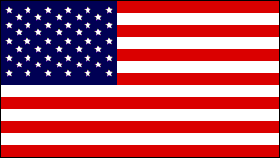RACISM IS EVIL AND UNAMERICAN!
updated 2001-04-14

# Pentominoes

Pentominoes are one of those simple ideas which turn out to be amazingly complex. They were re-popularized by Solomon Golomb in 1953 in a talk to the Harvard Math Club. In his book 'Polyominoes' (copyright 1965), though, Mr Golomb says that they were discussed heavily in the 1930s - 1940s in "Fairy Chess Review" (a British puzzle magazine), and that the underlying concept goes back to ancient times and the masters of Go. The first known pentomino problem was in "Canterbury Puzzles" in 1907.

____________________________________

It's a simple idea, really. Take 5 squares. Now look at all the possible combinations of these squares, such that each square touches at least one other square along a complete edge. There are exactly 12 such configurations, and, amazingly enough, each one looks like a letter of the alphabet. (OK, the F and the N require a little imagination.)

Here's the 12 letters: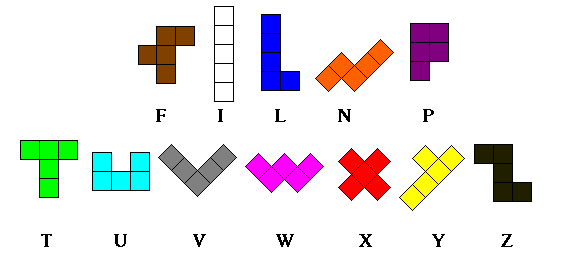(The coloring scheme is my own. I've played with them so long that these seem like the only appropriate colors, but that's just me.)

So what's so interesting about this? Consider: There are 12 letters, each of which is made up of 5 squares, giving a total of 60 squares. The challenge, then, is to arrange the letters into a 6 x 10 rectangle. Sounds easy enough until you try it. It turns out there are 2339 different ways to make a 6 x 10 rectangle! Here's three of them: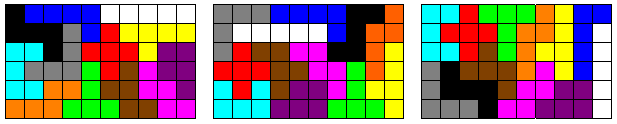The first solution above is interesting because it is actually 20 different solutions. Notice the T and the F make a symmetric pair; that is, you can pull them out, turn the pair around, and put it back in. The P and Y also make a symmetric pair. The I makes a symmetric pair with the P -Y combination; and the Z, U, and L make a symmetric group. Finally, the P and W make a symmetric group when the P isn't traded with the Y.

I really didn't like finding the second solution above. For a long time I had a theory that the I had to be on one of the edges. So much for that idea.

The third solution above is the second one I ever found. Unfortunately, I don't remember my first one.
When I first made a set of pentominoes, I sat down to play with them and had a 6 x 10 solution within a couple of minutes. "Hah!", I thought to myself, "this isn't so hard. Let's find a couple more." So I mixed up the letters and started again. Three hours later, I hadn't found another solution, and couldn't remember the first one, and had to get to sleep. The next night I tried again, and 4 hours later went to bed no closer to an answer. The next night, I discovered this answer and immediately memorized it. I'll never forget it.

As you might guess, it's also possible to make a 5 x 12 rectangle and a 4 x 15 rectangle. These are both somewhat easier than the 6 x 10, for some reason.
The 3 x 20 rectangle is especially challenging. It turns out there are exactly 2 solutions to this problem, and once you've found one, you also have the other.
You can also make an 8 x 8 square with the central 2 x 2 square missing, or an 8 x 8 square with its four corners missing, or lots of other shapes.

Another interesting challenge is the "triplication" puzzle: Choose one of the twelve pieces, and then use 9 of the others to form a copy of the chosen piece, 3 times as large in each dimension.

Also quite interesting are the "fence" problems, where you arrange the 12 pentominoes so as to enclose an empty space. (The pentominoes must touch along edges, not just corners.)
How large an area can you fence in if the empty space forms a rectangle, but the pentominoes border is freeform? (I've found an answer of size 90.)
A freeform space in a rectangular border? (I've found one of size 61.)
A rectangular space in a rectangular border? (I've found one of size 28.)
A freeform space in a freeform border? (I've found one of size 128.)

____________________________________

#### Try it yourself

I've written a Visual Basic program that lets you play with the pentominoes. Download it and let me know what you think. It's free.

The newest version is 3.5. If you have an earlier version, you really should upgrade. I've fixed several subtle bugs and made the design option easier to deal with.

Features:

� 18 pre-defined answer grids to solve, some of which you can modify for additional challenges
� the Triplication puzzle, mentioned above, for 12 more challenges
� a Design option, which lets you create your own puzzles by arranging the answer grid in whatever shape you want. You can click and drag to "rubber band"-select and move large sections of the answer grid.
� a Freeform option, which lets you play with the pentominoes without any defined answer grid
� an AutoSolve button, which will find a solution from a given starting point. AutoSolve can find more than one solution, or even search out all possible solutions.
� an option to not show progress during AutoSolve, which makes it much faster (but less fun)
� an option to play the competitive game (see below), with three levels of difficulty, using 15 different playing boards
� the ability to save your work in files for later display (a samples file is included)
� (32 bit version only) an option to be able to open these files by double-clicking on them.

32 bit (For Windows 95 or later)
The program is here: Pentominoes 3.5 (32bit) (zipped, 67K)
You also need a bunch of support files for this version, but you probably already have them. Search your computer for files named comdlg32.ocx and olepro32.dll . If they aren't there, you'll need to download the VB4 support files (zipped, 802K(!)).
And all VB4 programs need the file vb40032.dll, which should be in your /windows/system directory. You probably have it already, but if not, it is available from many places on the Web. Try any of the popular shareware Web pages, or just use a search page to find it. Here is a link to download it from Microsoft. (Get the Version 4 file.)

16 bit (For Windows 3.x users)
The program is here: Pentominoes 3.5 (16bit) (zipped, 56K)
It requires a support file, named vbrun300.dll , which should be in your /windows/system directory. If you don't have it, it's widely available. Any of the Web search engines should be able to find it for you. It's on the Win95 CD, too. Here is a link to download it from Microsoft. (Get the Version 3 file.)

I'm seriously thinking of writing a 3D version of this program (see below), but it's looking difficult so I might have to make it not free.

____________________________________

#### Pentominoes as a Game

The first place I read about pentominoes was Arthur C. Clarke's book "Imperial Earth", where they are used in a subplot.

In the afterword of the book, Mr. Clarke mentions that Mr. Golomb invented a game based on pentominoes. This game has very simple rules (players alternate placing a piece on an 8x8 square; the last one to play a piece wins), but is quite challenging.
There are 347 different starting plays, and over 1600 responses for most of them (I think). Games usually last about 8 plays, and so don't take much time. It is an interesting game.

Note: Hilarie Orman, while at the University of Arizona, proved that, on an 8x8 board, the game is a win for the first player. (I didn't use this fact in my program.)

____________________________________

#### Solid Pentominoes

I made my first set of pentominoes out of cardboard, and they quickly got thrashed. The next set I made out of wooden cubes, which gave me an idea. Since there are 60 squares (cubes), is it possible to make a 3 x 4 x 5 box?

It turns out it is. For example: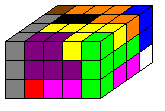I found about thirty solutions, and then wrote a program to search for others. It found 3940 of them!
There are many other interesting shapes you can make with the solid pentominoes. For example, you can make a scale model of each of the letters (except W and X), twice as long and twice as wide, three units high.

____________________________________

There was an article about pentominoes in the May 1957 edition of Scientific American. They also have appeared many times since then in the Mathematical Games column of that magazine.

Solomon Golomb's book "Polyominoes" has quite a bit of information about pentominoes. There is now a second edition of this book. (Princeton University Press; ISBN 0-691-08573-0)

The popular computer game Tetris is basically the same concept as pentominoes, but with 4 squares instead of 5.

The old Soma Cube puzzle was a 3D, 4-cube version. Actually, it didn't use all the possible combinations.

Phillipe Laurent has a Pentominoes page which goes into detail on the triplication problem.

The students of T.I.D. - Ronse school in Belgium have a nice Pentominoes page with a clever Excel spreadsheet to do the fence puzzle. They also have occasional pentominoes competitions, where you are challenged to find the best solution to a puzzle.

____________________________________

#### Rotations and Reflections

(for the more theoretical-minded)

Just how many solutions are there to the 3 x 4 x 5 puzzle?
That turns out to be an interesting question, and not quite as easy to answer as you might think.

First, to establish some terminology:
Consider the 3 x 4 x 5 solution above. Call the axis that is 5 boxes long the x-axis, call the 4-box axis the y-axis, and call the 3-box axis the z-axis.
Assign the coordinates (1,1,1) to the completely hidden corner in the above picture, and number the rest from there, so that the top of the T is at coordinates (5,4,3) (the completely visible corner above).
Now, number the 12 pentominoes in alphabetical order, so that F=1, I=2, L=3, ..., Z=12. Notice that each of the pieces also can be oriented several different ways. The F and P each have 24 possible orientations; the L, N, and Y each have 16 possible orientations (it would be 24 if the puzzle weren't 3 units high); the symmetric letters T, U, V, W, and Z each have 12; the doubly-symmetric X has 3; and the doubly-symmetric I has only 1 (it would be 3 if the puzzle were 5 units in each dimension).

Consider the 3 x 4 x 5 solution on the left below. If we pick it up, flip it around the x-axis, and put it back down, it becomes the solution on the right below.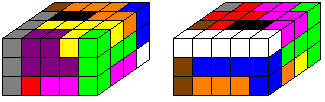Are these different solutions?
Every letter is in a different place or orientation, but obviously these can't really be called "different" solutions. Similarly, any combination of rotations about any axes won't make a "different" solution. So, to properly count the number of solutions to the 3 x 4 x 5 puzzle, we have to skip ones that are simple rotations of other solutions. Is there a way to identify these duplicate solutions?

One seemingly easy way is to fix the location of the I. Since it's 5 units long, the only way it will fit is lined up along the x-axis, which means there's only 12 possible places it can go. We've already seen that the I going from (1,1,1) to (5,1,1) is equivalent to having it go from (1,4,3) to (5,4,3); one more rotation will also show that the other two corners are also equivalent. By a suitable combination of rotations, the 12 possibilities can be reduced to 4 distinct ones: One end of the I must be at (1,1,1), (1,2,1), (1,1,2), or (1,2,2).

The solutions thus can be grouped into four categories, based on where the I is located.

Categories three and four, with the I in the middle layer (z=2), require a slight refinement, however.
Notice that for these solutions:
A rotation about the x-axis moves the I to (1,4,2) for category three, and (1,3,2) for category four. Both of these are not valid positions, but a rotation about the z-axis corrects that. In either case, the piece at (1,1,1) is traded with the piece at (5,1,3).
A rotation about the y-axis leaves the I in place, but switches the top and bottom layers. (Actually, the I is flipped, but it's the same as if it didn't move.) Again the piece which was at (1,1,1) gets moved to (5,1,3) and vice versa.
A rotation about the z-axis moves the I to (1,4,2) for category three, and (1,3,2) for category four. Both of these are not valid positions, but a rotation about the x-axis corrects that. In either case, the piece at (1,1,1) is traded with the piece at (5,1,3).

Thus a distinct solution in category three or four will have two possible orientations, which are equivalent by a rotation about the y-axis.

Since it is impossible for a single pentomino to occupy both (1,1,1) and (5,1,3), one of these two orientations will have a smaller-numbered piece at (1,1,1). Let's say that this is the 'true' orientation of the solution.

Our rule for eliminating simple rotations is thus:

1. The I must be located at (1,1,1), (1,2,1), (1,1,2), or (1,2,2).
2. If the I is in the middle layer, the piece at (1,1,1) must be alphabetically smaller than the piece at (5,1,3).

Now a more tricky problem.

Consider the two solutions below. Notice that the solution on the right is a mirror image of the solution on the left.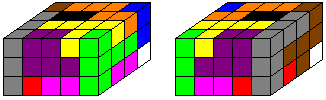Are these different solutions?
Again, the letters are all in different places or orientations, but again it seems wrong to call these "different" solutions. Notice, though, that the solution on the right cannot be made by any combination of rotations of the solution on the left. Our trick of fixing the location of the I doesn't really skip all the duplicate solutions.

Is there a way to tell if a solution is a combination of reflections and/or rotations of another solution?

Let's examine each of the four categories:

Consider a solution S of the first category, with the I at (1,1,1); and in particular notice the piece at (1,2,1) (F in the solution on the left above) as various reflections are applied.

When S is reflected through the xz plane to make a new "solution" S', the I moves to (1,4,1). This is not one of the four valid positions, so we must rotate S' about the z axis. Call the result S''. S'' is thus equivalent to S by reflection. Notice that the piece at (1,2,1) in S has moved to (5,2,1) in S''.

When S is reflected through the yz plane to make a new "solution" S', the I remains at (1,1,1). S' is equivalent to S. Notice that the piece at (1,2,1) in S has moved to (5,2,1) in S'.

When S is reflected through the xy plane to make a new "solution" S', the I moves to (1,1,3). This is not one of the four valid positions, so we must rotate S' about the y axis. Call the result S''. S'' is thus equivalent to S. Notice that the piece at (1,2,1) in S has moved to (5,2,1) in S''.

This means that any odd number of reflections is equivalent to a single reflection through the yz plane. (An even number of reflections yields the original solution.) Thus, each solution in category one has two orientations that are equivalent by reflection.
Since it is impossible for a single pentomino to occupy both (1,2,1) and (5,2,1) (except the I, which by definition is placed elsewhere), one of the two orientations will have a smaller-numbered piece at (1,2,1). Let's say this is the 'true' orientation.

Thus, if we specify that the piece at (1,2,1) must be smaller alphabetically than the piece at (5,2,1), we can eliminate all solutions in the first category which are reflections of another solution.

For category two (the I at (1,2,1)), the same argument holds, except that the locations to be compared are (1,1,1) and (5,1,1).

Categories three and four again require further restriction.
As above, reflection through the xz plane followed by a rotation about the z axis leaves the I in place and moves the piece at (1,1,1) to (5,1,1).
Also as above, reflection through the yz plane leaves the I in place and moves the piece at (1,1,1) to (5,1,1).
Now, though, reflection through the xy plane leaves the I in place, and moves the piece at (1,1,1) to (1,1,3). A rotation about the y-axis moves the piece to (5,1,1), but is not required to keep the I in a valid position.

Thus, solutions in these categories have three orientations which are equivalent by reflection (and a fourth by rotation, as shown above). The piece which is at (1,1,1) in one of the orientations is mapped to the other three corners of the xz plane in the others.
Since we've eliminated one of these orientations by saying the piece at (1,1,1) must be smaller than the one at (5,1,3), it makes sense to extend the rule by also eliminating all orientations where the piece at (1,1,1) is not the smallest of the four corners in that plane.

While it is impossible for a pentomino to occupy (1,1,1) and (5,1,1) (except the I, which by definition is placed elsewhere), it is possible for one pentomino to occupy (1,1,1) and (1,1,3). This happens with the U in category three, and with the P, T, U, and V in category four. These solutions, then, require further restriction.

The problem with these solutions is that after a reflection through the xy plane, they appear to still meet the qualifications for a true solution: The piece at (1,1,1) is still the smallest of the four corners. Notice, though, that this reflection trades the pieces at (5,1,1) and (5,1,3), so again we can distinguish them by choosing the one with the smaller piece at (5,1,1).

Naturally the question arises: What if the piece at (1,1,1) is also at (1,1,3) AND the piece at (5,1,1) is at (5,1,3)? This can happen in category 4, since the P, T, U, and V could each potentially occupy two corners.
Indeed there are solutions of this form. The logical extension of the rule is then to proceed to the other corners, and specify that the piece at (1,4,1) must be smaller than that at (1,4,3); and, if necessary, that if these are equal then the piece at (5,4,1) must be less than the one at (5,4,3).
Fortunately, there are no solutions (according to my program) in category 4 which have all four sets of corners matching. There is however, a solution with three sets: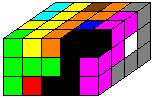To recap, then:

When counting distinct solutions to the 3 x 4 x 5 puzzle, we must skip any which are equivalent to some other solution by a combination of rotations and reflections. One way to do this is to label a solution as valid only if these criteria are met:

1. The I must occupy one of these four positions: (1,1,1), (1,2,1), (1,1,2), or (1,2,2).

2. a) For the first category, the piece at (1,2,1) must be alphabetically smaller than the piece at (5,2,1).
b) For the second category, the piece at (1,1,1) must be smaller alphabetically than the piece at (5,1,1).
c)For the third and fourth categories, the piece at (1,1,1) must be less than or equal to the pieces at (1,1,3), (5,1,1), and (5,1,3). If the piece at (1,1,1) is also at (1,1,3), the piece at (5,1,1) must be less than or equal to the piece at (5,1,3). If equal, the piece at (1,4,1) must be less than or equal to the piece at (1,4,3). If equal, the piece at (5,4,1) must be less than the piece at (5,4,3).

According to my program, the count of distinct solutions is:
 Category 1: 2715 Category 2: 358 Category 3: 337 Category 4: 530

for a total of 3940 different solutions.

Pretty amazing how far astray you can go starting with just 5 squares, isn't it?

____________________________________

copyright 1996 - 2000 by Ken Hinds